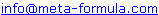# Metastock Formulas - D 1 Click here to go back to Metastock Formula Index

 IMPORTANT: These formulas aren't my complete collection. For my complete collection of instantly usable, profitable and powerful MetaStock formulas Click Here

Would You Like To Use MetaStock To Its Full Potential? Discover the Amazingly Simple Secret to Master Metastock Step-By-Step - click here

A | A 1 | B | B 1 | C | C 1 | D | D 1 | E | F | G | H | I | J | K | L | M | M 1 | N | O | P | R | S | S 1 | T | U | V | W | Z |

 Darvis Box - Based on "How I made 2 million on the stockmarket" Dr Elder's Force Index - Metastock Indicator Formula Date filter Divergences - Class A

## Darvis Box - Based on "How I made 2 million on the stockmarket"

Jason Prestwidge

Periods:=Input("periods",1,260,260);

Topbox:=If(Ref(H,-3)>=Ref(HHV(H,Periods),-4) AND Ref(H,-2)<Ref(H,-3) AND Ref(H,-1)<Ref(H,-3) AND H< Ref(H,-3),Ref(H,-3),PREVIOUS);

Botbox:=If(Ref(H,-3)>=Ref(HHV(H,Periods),-4) AND Ref(H,-2)<Ref(H,-3) AND Ref(H,-1)<Ref(H,-3) AND H< Ref(H,-3),LLV(L,4),PREVIOUS);

Topbox;
Botbox;

(Go Top...)

## Dr Elder's Force Index - Metastock Indicator Formula

FI:=(C-Ref(C,-1))*V;Mov(FI,13,E)
{Smoothed by 13 period exponential moving average}

Dr Elder also refers to a 2 period Force Index in which case, you would change the ‘13’ above to ‘2’.

(The following explanatory notes are taken from ‘Trading for a Living’ by Dr. Alexander Elder, Published by John Wiley & Sons, Inc, 1993)

“Force Index is an oscillator developed by this author. It measures the force of bulls behind every rally and of bears behind every decline.

Force Index combines three essential pieces of market information – the direction of price change, its extent, and trading volume. It provides a new, practical way of using volume to make trading decisions.

Force Index can be used raw, but it works better if you smooth it with a moving average. Force Index smoothed with a short MA helps pinpoint entry and exit points. Force Index smoothed with a long MA reveals major changes in the force of bulls and bears.

A 2-day EMA of Force Index provides a minimal degree of smoothing. It is useful for finding entry points into the markets. It pays to buy when the 2-day EMA is negative and sell when it is positive, as long as you trade in the direction of the 13-day EMA of prices.

A 13-day EMA of Force Index tracks longer term changes in the force of bulls and bears. When it crosses above its centerline, it shows the bulls are in control. When it turns negative, it shows that bears are in control. Divergences between 13-day EMA of Force Index and prices identify important turning points.”

(Go Top...)

## Date filter

{ Date filter }
{ Plots +1 signal within user-input date period }
{ [email protected] }

StDay:=Input("start Day",1,31,1);
StMnth:=Input("start Month",1,12,1);
StYear:=Input("start Year",1800,2200,2003);
EnDay:=Input("end Day",1,31,31);
EnMnth:=Input("end Month",1,12,12);
EnYear:=Input("end Year",1800,2200,2003);

start:=Year()>StYear
OR (Year()=StYear AND (Month()>StMnth
OR Month()=StMnth AND DayOfMonth()>=StDay));
end:=Year()<EnYear
OR (Year()=EnYear AND (Month()<EnMnth
OR Month()=EnMnth AND DayOfMonth()<=EnDay));

start AND (end OR (start AND Alert(start=0,2)))

(Go Top...)

## Divergences - Class A

{ Class A price/indicator divergence v3 }{ +1 signal = bullish trough divergences }{ -1 signal = bearish peak divergences }{ ©Copyright 2003 Jose Silva }{ [email protected] }

ind:=Input("MACD=1,  RSI=2,  DMI=3,  Mom=4",1,4,1);
pds:=Input("indicator periods",2,2520,10);
f:=Input("price field: Close=1, High/Low=2",1,2,1);
Ch:=Input("peak/trough depth minimum (0-100%)",0,100,0)/100;
shift:=Input("shift signals back to match divergences=1",0,1,0);

{y:=insert any indicator or Fml("formula name")}
y:=If(ind=1,MACD(),If(ind=2,RSI(C,pds),
If(ind=3,DMI(C),Mo(C,pds))));

xu:=If(f=1,C,H);
xd:=If(f=1,C,L);
Pkx:=xu<Ref(xu,-1) AND Ref(xu,-1)>Ref(xu,-2)
AND Ref(xu,-1)>=(xu+Ref(xu,-2))/2*(1+Ch);
Pkx1:=ValueWhen(1,Pkx,Ref(xu,-1));
Pkx2:=ValueWhen(2,Pkx,Ref(xu,-1));
Trx:=xd>Ref(xd,-1) AND Ref(xd,-1)<Ref(xd,-2)
AND Ref(xd,-1)<=(xd+Ref(xd,-2))/2*(1-Ch);
Trx1:=ValueWhen(1,Trx,Ref(xd,-1));
Trx2:=ValueWhen(2,Trx,Ref(xd,-1));

Pky:=y<Ref(y,-1) AND Ref(y,-1)>Ref(y,-2)
AND Ref(y,-1)>=(y+Ref(y,-2))/2*(1+Ch);
Pky1:=ValueWhen(1,Pky,Ref(y,-1));
Pky2:=ValueWhen(2,Pky,Ref(y,-1));
Try:=y>Ref(y,-1) AND Ref(y,-1)<Ref(y,-2)
AND Ref(y,-1)<=(y+Ref(y,-2))/2*(1-Ch);
Try1:=ValueWhen(1,Try,Ref(y,-1));
Try2:=ValueWhen(2,Try,Ref(y,-1));

0;
Ref(Trx AND Try AND Trx1<Trx2
AND Try1>Try2,shift)
-Ref((Pkx AND Pky AND Pkx1>Pkx2
AND Pky1<Pky2),shift)

(Go Top...)

If you have Metastock formulas you would like to share,
Please email toWe look forward to hearing from you!